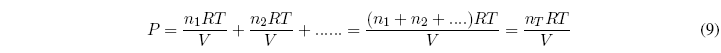In 1810 Dalton established that the total pressure of a mixture of gases is equal to the sum of the pressures that each gas would exert if it were alone in the container.
Dalton's Law: "The sum of the partial pressures of each gas is equal to the total pressure of the gas mixture."

Let be a mixture of $n_1, n_2....$ moles of gases. The total pressure can be calculated with the equation:Another way of expressing Dalton's law, very useful in solving problems is: $P_i =x_iP$ , where $P_i$ is the partial pressure of gas i, $x_i$ is the mole fraction of gas i in the mixture of gases and P is the total pressure.